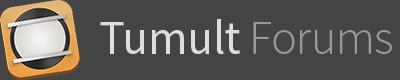# Display Percent by Time Duration

I have redone my original Percent Count down project.

To a version that uses a Symbol which is controlled and configured using JS. ( Only counting up )

The JS code is small but easy to configure for each instance of the Symbol.
Each Instance of the Symbol is given a a Unique Element ID ( using the inspector )

Each Symbol instance is started on the Main Timeline of the Project by it’s own Symbol Action. ( Start Timeline ‘Main Timeline’ )

In the JS.

You configure for each Instance using simple if clauses .

`````` //----  Set up per display
var timeToCount, maxPercent;

if (element.id ==  'percent100' ) {

timeToCount = 10; //---seconds
maxPercent = 100; //---max Percent
}

if (element.id ==  'percent75' ) {

timeToCount = 5; //---seconds
maxPercent = 75; //---max Percent
}
``````

Notice I just get each Symbol Instance’s id using element.id. This is because the id is now passed along when the Symbol Action fires. ( Brilliant)

The JS will be run by all of the Symbol Instance you have given a Symbol Action to run. because the Symbol has its own Action on it’s main timeline to run the Javascript.

Now that we have configured for each Symbol instance. Each one will only run the rest of the JS using it’s settings.

``````var thisWidth = 0;  //-- width increment
var countDownTimerWidth =  \$('#'+ element.id +' .countDownTimer1').width(); //-- get width of bar.

var thisFire =   setInterval(function(){

thisWidth += (countDownTimerWidth /100) ; //-- workout increment size

var thisPercent = Math.floor((thisWidth/ countDownTimerWidth * 100 )); //-- calculate  current percentage of bar

if (thisPercent >=  maxPercent) { //-- stop at pecentage
clearInterval( thisFire);

}

\$('#'+ element.id +' .countDownTimer1').html("%" + thisPercent)  ; //-- set progress text

\$('#'+ element.id +' .percentBar').width(thisWidth); //-- set progress bar width

}, timeToCount * 10) ;
``````

(
I have used JQuery with this project, originally I did it all in native JS but it is much simpler to get individual class instances with JQuery than it is with JS, where you would need more repeating code and have to use indexes . i.e 
)

This example has two instances. One set to stop at 75% and run in 5 seconds. The Other to Stop at %100 and run in 10 seconds2 Likes

This is the UP or Down Version.

Which means in the config section of the JS you can set which direction the bar will move and count.

``````if (element.id ==  'percent100' ) {

timeToCount = 10; //---seconds

maxPercent = 15; //---end max Percent
countDirectionUp = true;
}

if (element.id ==  'percent75' ) {

timeToCount = 10; //---seconds
maxPercent = 25; //---end max Percent
countDirectionUp = false;
}
``````

I have used ternary operators and the countDirectionUp as the condition. Thank god for ternary operators .

Full code:

``````	 //---- CONFIG -  Set up per display  -- Percents are always measured  from  0 - 100
var timeToCount, maxPercent,countDirectionUp, thisWidth;

if (element.id ==  'percent100' ) {

timeToCount = 10; //---seconds

maxPercent = 15; //---end max Percent
countDirectionUp = true;
}

if (element.id ==  'percent75' ) {

timeToCount = 10; //---seconds
maxPercent = 25; //---end max Percent
countDirectionUp = false;
}

//-- Full width
var countDownTimerWidth =  \$('#'+ element.id +' .countDownTimer1').width(); //-- get width of bar.

thisWidth =  (countDirectionUp ?  0 :  \$('#'+ element.id +' .countDownTimer1').width()) //-- starting width of Bar.

var thisFire =   setInterval(function(){
//-- width increment
thisWidth =  (countDirectionUp ?  thisWidth += (countDownTimerWidth /100) :  thisWidth -= (countDownTimerWidth /100)); //-- workout increment size

var thisPercent = Math.floor((thisWidth/ countDownTimerWidth * 100 )); //-- calculate  current percentage of bar

if ((countDirectionUp ?  thisPercent >=  maxPercent :  thisPercent <=  maxPercent)) { //-- stop at pecentage

clearInterval( thisFire);

}

\$('#'+ element.id +' .countDownTimer1').html("%" + thisPercent)  ; //-- set progress text

\$('#'+ element.id +' .percentBar').width(thisWidth); //-- set progress bar width

}, (countDirectionUp ?  (timeToCount * 1000) / maxPercent :  timeToCount*1000  / ( 100 - maxPercent)) ) ;
``````
1 Like

Thanks for sharing Mark! Would love to start a thread in here called Hype Toolbox which is a collection of the most useful scripts, techniques and workarounds for Hype and add things like this to it.

oops, I see there is a template gallery in here already.

Thats what the Template Galley Category is partially for…

There ar other Cats for javascript etc. But you cannot really post every individual part in the individual cats.

I.e the JS tricks in this project could go into the Javascript one but I think if you do that then things could become a mess.